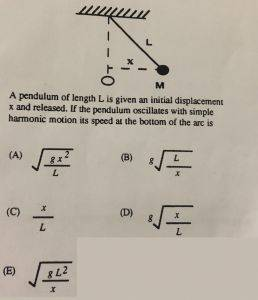# Speed of a pendulum at the bottom

Fontseeker

## Homework Statement## Homework Equations

U = (1/2)kx^2
T = 2 * π * (m/k)^ (1/2)

## The Attempt at a Solution

[/B]
I said that the angular velocity is

w = (2 * π) / t

And the equation for velocity would be:

v(t) = -Aw * cos (w * t)

However, I don't know how to relate this to the speed at the bottom. I was thinking of using conservation of energy, but I don't have the height.

#### Attachments

Dr Dr news
The conservation of energy will work. The potential energy + the kinetic energy = the total energy is a constant. If you take the potential energy as zero at the bottom of the path, the total energy at that point is all kinetic energy. If you start from rest, the total energy at the start is all potential energy. So the kinetic energy at the bottom is equal to the potential energy at the start. You know that the potential energy is mgh and the kinetic energy is mv^2/2 so all you need to do is determine what h is in terms of the parameters of the problem.

Homework Helper
Gold Member
2022 Award
I don't have the height.
Ask Pythagoras for help on that one.

Alternatively, you can rule out all but one of the answers quite quickly.

Fontseeker
Ask Pythagoras for help on that one.

Alternatively, you can rule out all but one of the answers quite quickly.

Alright, so if I use conservation of energy:

(1/2)mv^2 = mgh
v = (2gh)^(1/2)

The height would be h = (L^2 -x^2)^(1/2)
so the Velocity = (2g * (L^2 -x^2)^(1/2))^(1/2)

That doesn't show up on the answers.